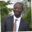Related Tags

communitycreator
java
calculator

# How to build a basic mortgage calculator in JavaAbel Lifaefi Mbula

### Overview

In this shot, we’ll learn to build a mortgage calculator in Java.

### Setting up and getting user inputs

First, let’s create our class with the starting point, the main method.

public class Mortgage {
public static void main(String[] args) {
}
}


The name for our class is Mortgage. This means that the file containing the source code will also be Mortgage.

Next, we can write the code to get user inputs inside the main method.

Scanner stdin = new Scanner ( System.in );

// Principal
System.out.print("Principal:");
int principal = stdin.nextInt();

// Interest rate
System.out.print("Annual Interest Rate: ");
float annualInterest = stdin.nextFloat();

// Period (max = 30)
System.out.print("Period (Years): ");
byte years = stdin.nextByte();

stdin.close();


We use the Scanner class to get the user input, which is defined in java.util. We import the class and create a new object, stdin.

These are the inputs we need from the user:

• principal: The amount of loan they want to get.
• Annual interest rate in percent.
• period in years: The maximum value is 30 (years).

So, to do this, we first print out the question like:

System.out.print("Principal:")


Note: We use System.out.print() instead of System.out.println(). This is because we want the user answer to be printed next to the question.

After the question, we create a variable and keep the user input. Syntax:

type varName = scannerObj.nextType()


Example.

float annualInterest = stdin.nextFloat()


### Implement the mortgage logic

To calculate the monthly payment of a mortgage, we use this formula:

$M=P \frac{r(1+r)^{n}}{(1+r)^{n}-1}$

• $M$: Monthly payment
• $P$: Principal
• $r$: Monthly rate (divide the annual rate by 12)
• $n$: Number of payments (the number of months you will be paying the loan)

Before calculating the mortgage monthly payment, let’s find the monthly rate and the number of payments.

float monthlyInterest = annualInterest / 100 / 12

int numberOfPayments = years * 12


Now, we can calculate the monthly payment.

double mathPower = Math.pow(1 + monthlyInterest, numberOfPayments);

double monthlyPayment = principal * (monthlyInterest * mathPower / (mathPower - 1));

String monthlyPaymentFormatted = NumberFormat.getCurrencyInstance().format(monthlyPayment);


First, we calculate 1 + r to the power of n and keep the value into the variable mathPower.

Next, we calculate the monthly payment and assign the value to monthlyPayment.

To format the monthly payment into US dollars, we take the help of NumberFormat. Therefore, we’ll first need to import it like this:

import java.text.NumberFormat


### Put all together and print the result

Let’s put all of the above together and run our code.

import java.util.Scanner;
import java.text.NumberFormat;

public class Mortgage {
public static void main(String[] args) {
Scanner stdin = new Scanner ( System.in );

// Principal
System.out.print("Principal:");
int principal = stdin.nextInt();

// Interest rate
System.out.print("Annual Interest Rate: ");
float annualInterest = stdin.nextFloat();
float monthlyInterest = annualInterest / 100 / 12;

// Period (max = 30)
System.out.print("Period (Years): ");
byte years = stdin.nextByte();
int numberOfPayments = years * 12;

stdin.close();

// Calculate the monthly payment
double mathPower = Math.pow(1 + monthlyInterest, numberOfPayments);

double monthlyPayment = principal * (monthlyInterest * mathPower / (mathPower - 1));

String monthlyPaymentFormatted = NumberFormat.getCurrencyInstance().format(monthlyPayment);

// Print the result
System.out.print("Your montly payment is: " + monthlyPaymentFormatted);
}
}
Code execution

### Improvement

The program above is fine but can still be improved.

Let’s define the numbers 100 and 12 as constants and give them proper names.

final byte MONTHS_IN_YEARS = 12;
final byte PERCENT = 100;


Next, we can replace those magic numbers with their variables.

Note: A final variable cannot change.

### Summary

Congratulations. You have coded your monthly mortgage calculator in Java. Let’s now recall the main point:

• Import packages: import package;
• Get user input: Scanner stdin = new Scanner ( System.in );
• format number: NumberFormat.getCurrencyInstance().format(number);

Happy coding!

RELATED TAGS

communitycreator
java
calculator

CONTRIBUTORAbel Lifaefi Mbula
RELATED COURSES

View all Courses

Keep Exploring

Learn in-demand tech skills in half the time